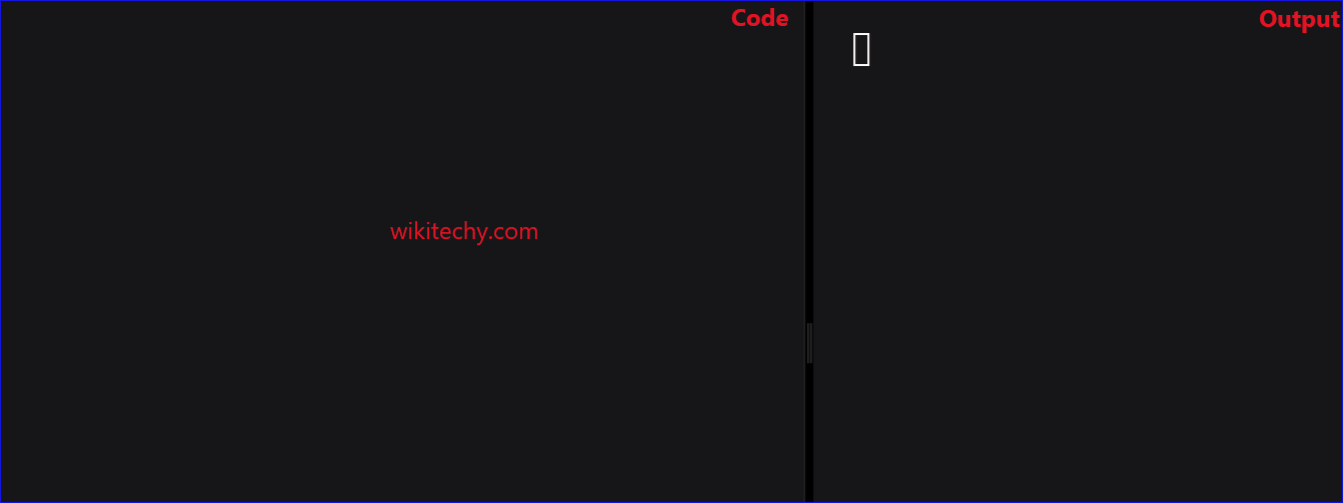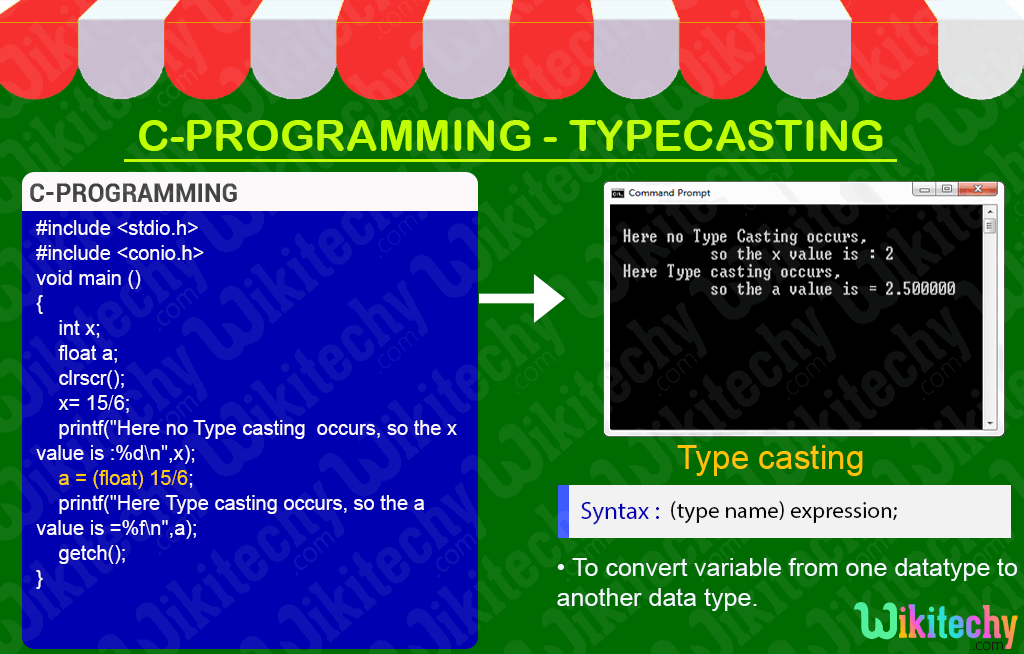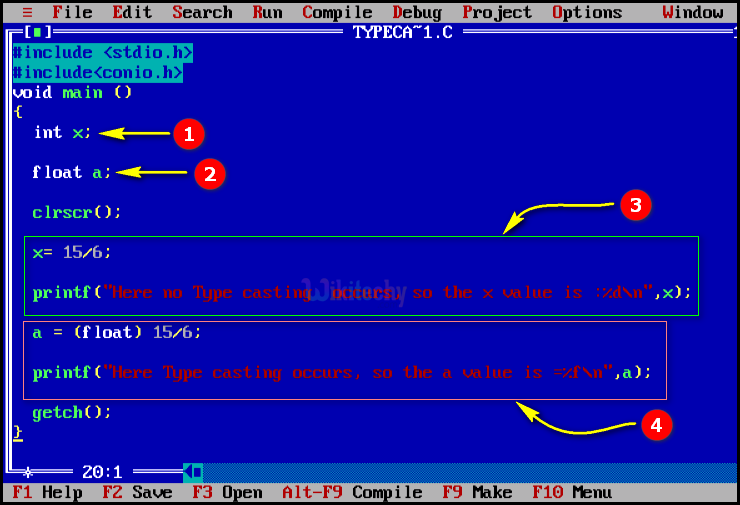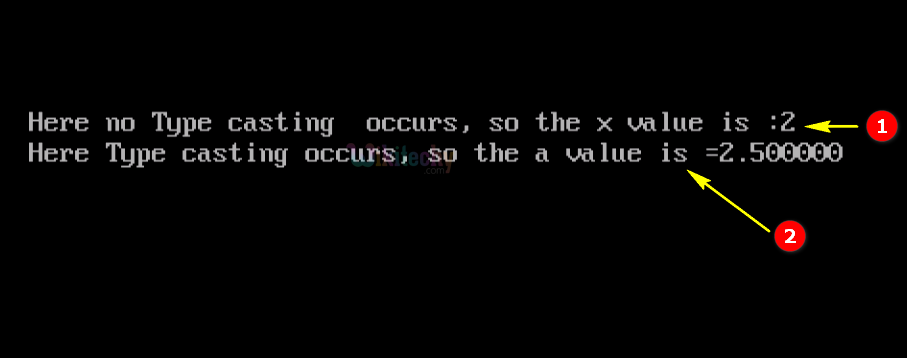# Typecasting in CLearn C - C tutorial - Typecasting in c - C examples - C programs

## Typecasting in C - Definition and Usage

• In C- Programming the type casting is used to convert the variable from one datatype to another data type, after we perform casting the variable datatype will be changed.
• “stdlib.h” header file supports all the type casting functions in C language programing.## Inbuilt typecast functions in C :

• In C-Programming some inbuilt functions are used.
• These inbuilt functions are used for defining the data type conversion from one type to another.
• And some of the inbuilt functions are listed below as follows,
1. atof() – Conversion of String to Float
2. atoi() – Conversion of String to Integer
3. atol() – Conversion of String to Long
4. itof() – Conversion of Integer to Float
5. ltoa() – Conversion of Long to String

## atof ( ) :

• In c-Programming atof() function is used for converting the variable data type from string data type to float data type .

## atoi() :

• In c-Programming atoi() function is used for converting the variable data type from string data type to int data type .

## atol() :

• In c-Programming atol() function is used for converting the variable data type from string data type to long data type .

## itos() :

• In c-Programming itos() function is used for converting the variable data type from integer data type to string data type .

## ltoa() :

• In c-Programming ltoa() function is used for converting the variable data type from long data type to string data type .

## C Code - Explanation1. In this statement we are declared the variable “X” as integer data type.
2. In this statement we are declared the variable “a” as float data type.
3. Here we perform a division operation and the output values will be in float (2.5000) which will be stored in the variable “x”. In the printf statement the integer output value as ”2” will be printed.
4. Here we perform the division operation and the output value will be in float (2.5000) which will be stored in the variable “a”, the printf statement will print the output value in float.

## Output :1. Here in this output the “x” value (2) will be printed with its printf statement “Here no Type casting occurs, so the x value is:” in the console window.
2. Here we print the float value of the variable “a” =2.500000 with its printf statement “Here Type casting occurs, so a value is “in the console window.